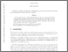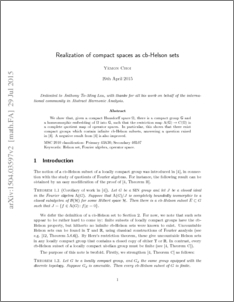# Realization of compact spaces as cb-Helson sets

Choi, Yemon (2016) Realization of compact spaces as cb-Helson sets. Annals of Functional Analysis, 7 (1). pp. 158-169. ISSN 2008-8752Preview
PDF (1504.03597v2)
1504.03597v2.pdf - Accepted Version
We show that, given a compact Hausdorff space $\Omega$, there is a compact group ${\mathbb G}$ and a homeomorphic embedding of $\Omega$ into ${\mathbb G}$, such that the restriction map ${\rm A}({\mathbb G})\to C(\Omega)$ is a complete quotient map of operator spaces. In particular, this shows that there exist compact groups which contain infinite cb-Helson subsets, answering a question raised in [Choi--Samei, Proc. AMS 2013; cf. http://arxiv.org/abs/1104.2953]. A negative result from the same paper is also improved.View Item